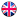EnglishHot keywords :

#### What is the Function of Capacitors in Circuits？

2019-08-23

Capacitors, usually referred to as capacitors, have the ability to accommodate charges. They are represented by the letter C. What is the role of capacitors in circuits? Capacitors are one of the most widely used electronic components in electronic devices. They are widely used in the fields of isolated direct traffic, coupling, bypass, filtering, tuning circuit, energy conversion, control and so on.

What is the Function of Capacitors in Circuits?

In DC circuits, capacitors are equivalent to circuit breaks. Capacitor is a kind of component that can store charge, and it is also one of the most commonly used electronic components.

However, in AC circuits, the direction of current varies with time as a function. The charging and discharging process of capacitors takes time. At this time, a variable electric field is formed between the plates, which is also a function of time. In fact, current passes through capacitors in the form of an electric field.

Coupling: Capacitor used in coupling circuit is called coupling capacitor. It is widely used in resistor-capacitor coupled amplifier and other capacitor coupled circuit to isolate DC and AC.

Filtering: The capacitor used in the filter circuit is called filter capacitor, which is used in power filter and various filter circuits. The filter capacitor removes the signal in a certain frequency band from the total signal.

Tuning Circuit: The capacitor used in the decoupling circuit is called decoupling capacitor, which is used in the DC voltage supply circuit of the multistage amplifier. The decoupling capacitor eliminates the harmful low frequency cross-connection between each stage amplifier.

High Frequency Vibration Absorption: The capacitance used in the high frequency vibration elimination circuit is called the high frequency vibration elimination capacitance. In the audio negative feedback amplifier, in order to eliminate the possible high frequency self-excitation of the vibration, this capacitance circuit is used to eliminate the high frequency whistle of the amplifier.

Resonance: Capacitors used in LC resonant circuits are called resonant capacitors, which are required in parallel and series LC resonant circuits.

Bypass: The capacitor used in bypass circuit is called bypass capacitor. If the signal of a certain frequency band needs to be removed from the signal in the circuit, bypass capacitor circuit can be used. According to the frequency of the removed signal, there are full frequency (all AC signals) bypass capacitor circuit and high frequency bypass capacitor circuit.

Balancing: Capacitors used in neutralizing circuits are called neutralizing capacitors. In radio and IF amplifiers and TV high frequency amplifiers, this neutralizing capacitor circuit is used to eliminate self-excitation.

Timing: The capacitor used in timing circuit is called timing capacitor. Timing capacitor circuit is used in the circuit which needs time control by capacitor charging and discharging. Capacitance plays the role of controlling time constant.

Integral: Capacitors used in integral circuits are called integral capacitors. In the synchronous separation circuit of electric field scanning, the field synchronization signal can be extracted from the field composite synchronization signal by using this integrated capacitance circuit.

Differential: Capacitors used in differential circuits are called differential capacitors. In order to get the tip trigger signal in flip-flop circuit, this differential capacitor circuit is used to get the tip trigger signal from all kinds of (mainly rectangular) signals.

Compensate: The capacitor used in the compensation circuit is called compensation capacitor. In the bass compensation circuit of the card holder, this low frequency compensation capacitor circuit is used to enhance the low frequency signal in the playback signal. In addition, there is a high frequency compensation capacitor circuit.

Bootstrap: The capacitor used in bootstrap circuit is called bootstrap capacitor. The output stage circuit of OTL power amplifier commonly uses this bootstrap capacitor circuit to raise the positive half-cycle amplitude of the signal in a small amount by means of positive feedback.

Frequency Dividing: The capacitor in the frequency dividing circuit is called the frequency dividing capacitor. In the loudspeaker frequency dividing circuit of the speaker, the frequency dividing capacitor circuit is used to make the high frequency loudspeaker work in the high frequency band, the medium frequency loudspeaker work in the middle frequency band, and the low frequency loudspeaker work in the low frequency band.

Load Capacitance: It refers to the effective external capacitance that determines the resonant frequency of the load together with the quartz crystal resonator. The common standard values of load capacitance are 16pF, 20pF, 30pF, 50pF and 100pF. Load capacitance can be adjusted appropriately according to the specific conditions. The working frequency of the resonator can generally be adjusted to nominal value by adjusting.

Capacitance Unit Conversion Formula

Capacitance of capacitors. A parameter describing the capacitance of a capacitor in the form of "Farad (F)". In practical application, capacitance appearing in "Farad" is seldom seen, and other expansion units commonly used and common are μF and pF.

1F=100mF=1,000,000μF(106μF)=1,000,000,000nF(109nF)=1,000,000,000,000pF(1012pF)

Green Tech manufacture and sell silver mica capacitors, tantalum capacitors, film capacitors, super capacitors and high reliability solutions for telecom, medical, new energy, rail, aviation, defense, space etc. We have served over 1000 customers around the world.